Euler's Method for systems of ordinary differential equations using Mathematica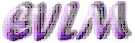Anotation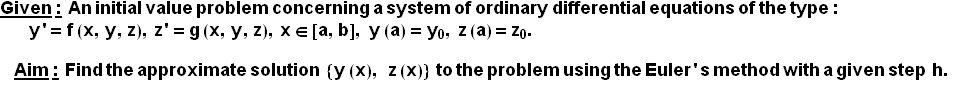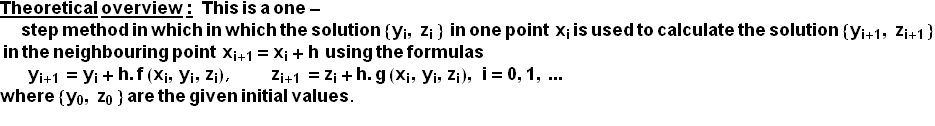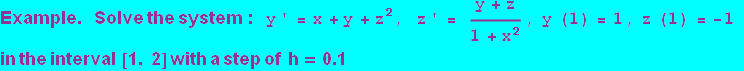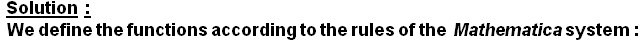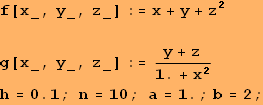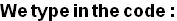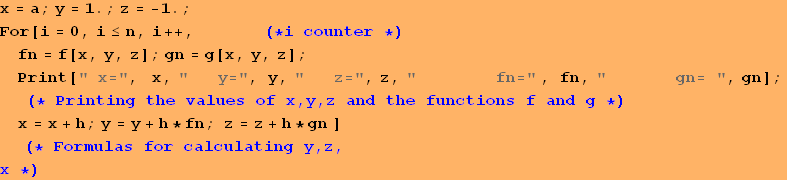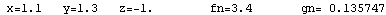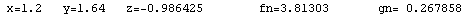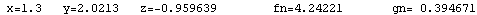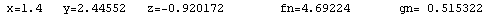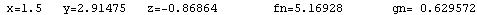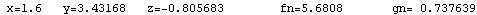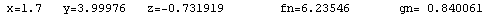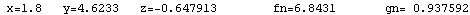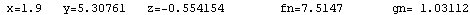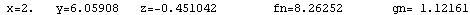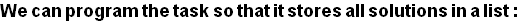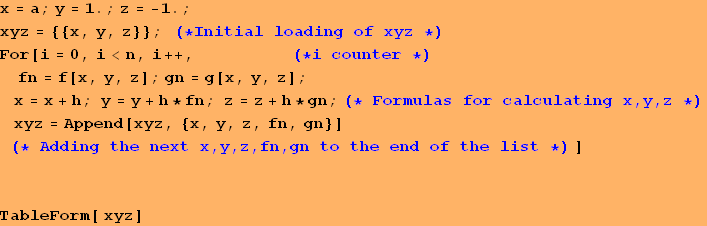1 1 -1 1.1 1.3 -1 3. 0. 1.2 1.64 -0.986425 3.4 0.135747 1.3 2.0213 -0.959639 3.81303 0.267858 1.4 2.44552 -0.920172 4.24221 0.394671 1.5 2.91475 -0.86864 4.69224 0.515322 1.6 3.43168 -0.805683 5.16928 0.629572 1.7 3.99976 -0.731919 5.6808 0.737639 1.8 4.6233 -0.647913 6.23546 0.840061 1.9 5.30761 -0.554154 6.8431 0.937592 2 6.05908 -0.451042 7.5147 1.03112

Conclusion:  The solution derived using the Euler's method is in the form of a table of the variable functions y(x), z(x), as shown in the second and third column of the table above, respectively.  Since the априорна error of the method is О(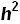) and here h=0.1, then these solutions must be taken rounded only to the second symbol after the decimal point.

Graphic of the solution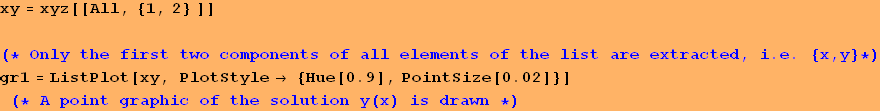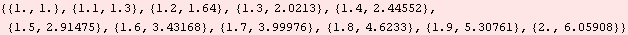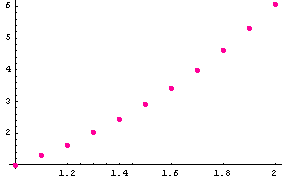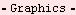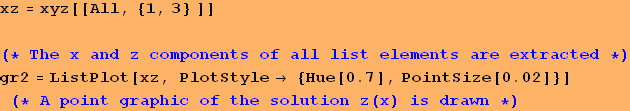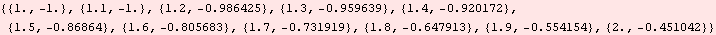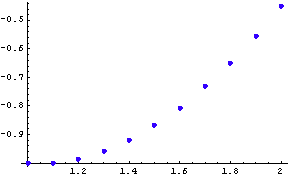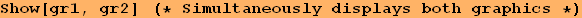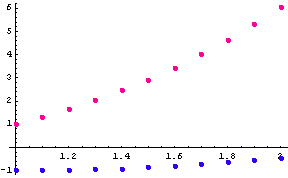Created by Wolfram Mathematica 6.0  (22 September 2008)